### Explanation of Classical Electron Diffraction

The Central Mystery of Quantum Mechanics

A gun (obeying classical physics) sprays bullets towards a target. Before they reach the target, they must pass through a screen with two slits. If bullets go through the slits they will most likely land directly behind the slit, but if they come in at a slight angle, they will land slightly to the sides. The resulting pattern is a map of the likelihood of a bullet landing at each point.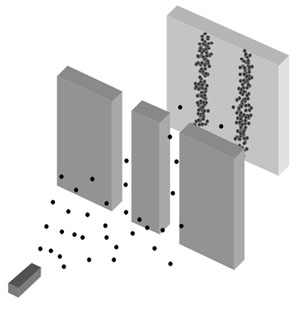The above two-slit pattern happens to be simply the sum of the patterns for each slit considered separately: if half the bullets were fired with only the left slit open and then half were fired with just the right slit open, the result would be the same.

With waves, however, the result is very different, because of interference. If the slits were opened one at a time, the pattern would resemble that for bullets: two distinct peaks. But when both slits are open, the waves pass through both slits at once and interfere with each other: where they are in phase they reinforce each other; where they are out of phase they cancel each other out.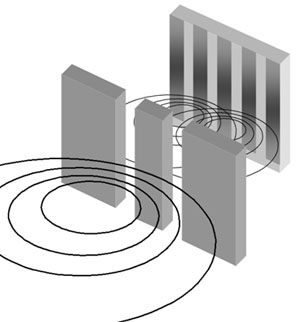Double Slit Experiment for Waves

Now the quantum paradox: Electrons, like bullets, strike the target one at a time. Yet, like waves, they create an interference pattern.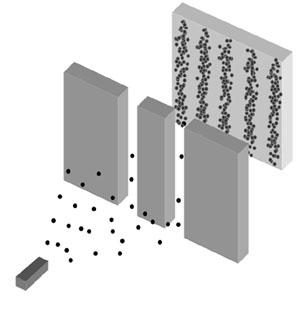Double Slit Experiment for Electrons

If each electron passes individually through one slit, with what does it “interfere?” Although each electron arrives at the target at a single place and time, it seems that each has passed through – or somehow felt the presence of both slits at once. Thus, the electron is understood in terms of a wave-particle duality.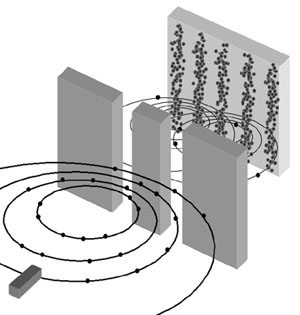Illustration of “Wave-Particle Duality”

The wave-particle duality is the central mystery of quantum mechanics–the one to which all others can ultimately be reduced.

### The Classical Solution

Click below to view animations.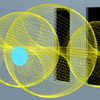Classical Electron DiffractionSimulation of the double-slit experiment with electrons. As the electron passes through the slits, it absorbs and emits photons, interacting with both slits. The photon far field pattern of the two-slit shape is imprinted on the electron beam pattern.The free electron is a plane lamina disk of charge obeying the de Broglie relationship that arises from Maxwell’s Equations (see the Classical Physics of the de Broglie Relationship section of Chapter 3 of R. Mills). As the free electron approaches the slits, its angular momentum vector (shown in black) is randomly oriented. The electron charge induces mirror charges on the slits; the resulting interaction causes the electron to become polarized so that the angular momentum vector is either parallel or antiparallel to the z-axis, the axis of propagation and the normal to the plane of the slits.

The interaction of the electron with the slits is mediated by mirror currents that form on the slits, and photons emitted from the currents. When a photon interacts with the electron, the angular frequency (de Broglie wavelength) of the electron changes, with the change matching the frequency of the photon (see Mills Chapter 3).

For essentially elastic diffraction, the energies are low and the photons are large, encompassing and emanating from both slits. Each photon has a quantized angular momentum of h-bar. The angular momentum vector of the electron precesses about the angular momentum vector of the absorbed photon.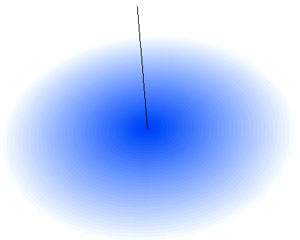Animation: Precession of the free electron about an axis.
Angular momentum vector shown in black.

As a result, some of the momentum is transferred from the z-axis to the transverse axis. Exactly how much depends on the strength and duration of the photon-electron interaction. The electron’s angular momentum vector is reoriented upon absorption and emission of one or more photons.

Over time, the incident electron beam statistically produces a uniform distribution across the slits. The photon pattern is also uniform across the slits (wherein the statistics is deterministic and local-causal, unlike the quantum mechanical case). Since the electron and each photon have quantized angular momentum in units of h-bar, the photon far-field pattern is imprinted on the electron-beam pattern over time. It is a transverse-momentum map given by the Fourier transform of the two slit shape; this arises classically due to conservation of power flow.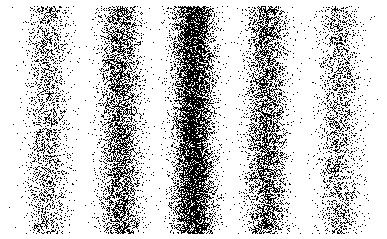The far field distribution.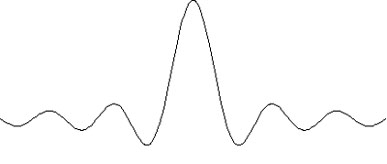Sinc Function

The amplitude of the sinc function is due to the periodic reversal of the electron angular momentum vector. The envelope amplitude is due to the decreasing probability of electrons landing farther out from center. These require a stronger electron-photon interaction to get a stronger transverse momentum transfer, but stronger interactions are less probable.

Since the number of electrons hitting a given position on the detector over time is proportional to the electron kinetic energy, the intensity pattern is the square of the amplitude. The predicted result is equivalent to the observed double slit interference pattern for waves.

Mystery Resolved

According to quantum mechanics, the electron is a point, but simultaneously everywhere at once, and it goes through both slits simultaneously, “guided” by a probability wave with a phase that depends on the position-momentum Heisenberg Uncertainty Principle: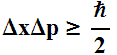where the phase contains the term Delta-p that is interpreted as the contribution to the uncertainty in the momentum of the incident particle on scattering. In the classical picture, the phase also contains the term Delta-p that is the physical momentum change of the incident particle on scattering. In both cases, the change in position, Delta-x, corresponds to the transverse displacement of the particle due to diffraction.

The electron only goes through one slit but is imprinted with the wave character of photons that are created across both slits due to electron-slit interaction. An electromagnetic wave exists. Quantum mechanics reproduces the mathematics that corresponds to this physical electromagnetic wave by invoking a nonsensical waving probability. Thus, it is stuck with the unfortunate result that the “wave-particle duality is unlike anything in our common everyday experience.”

Physics can now be reinstated over mysticism for this simple experiment with an understanding of the physical nature of fundamental particles. The details are given in Chapter 8 and Chapter 3 of R. Mills.

2005 Brilliant Light Power, Inc.